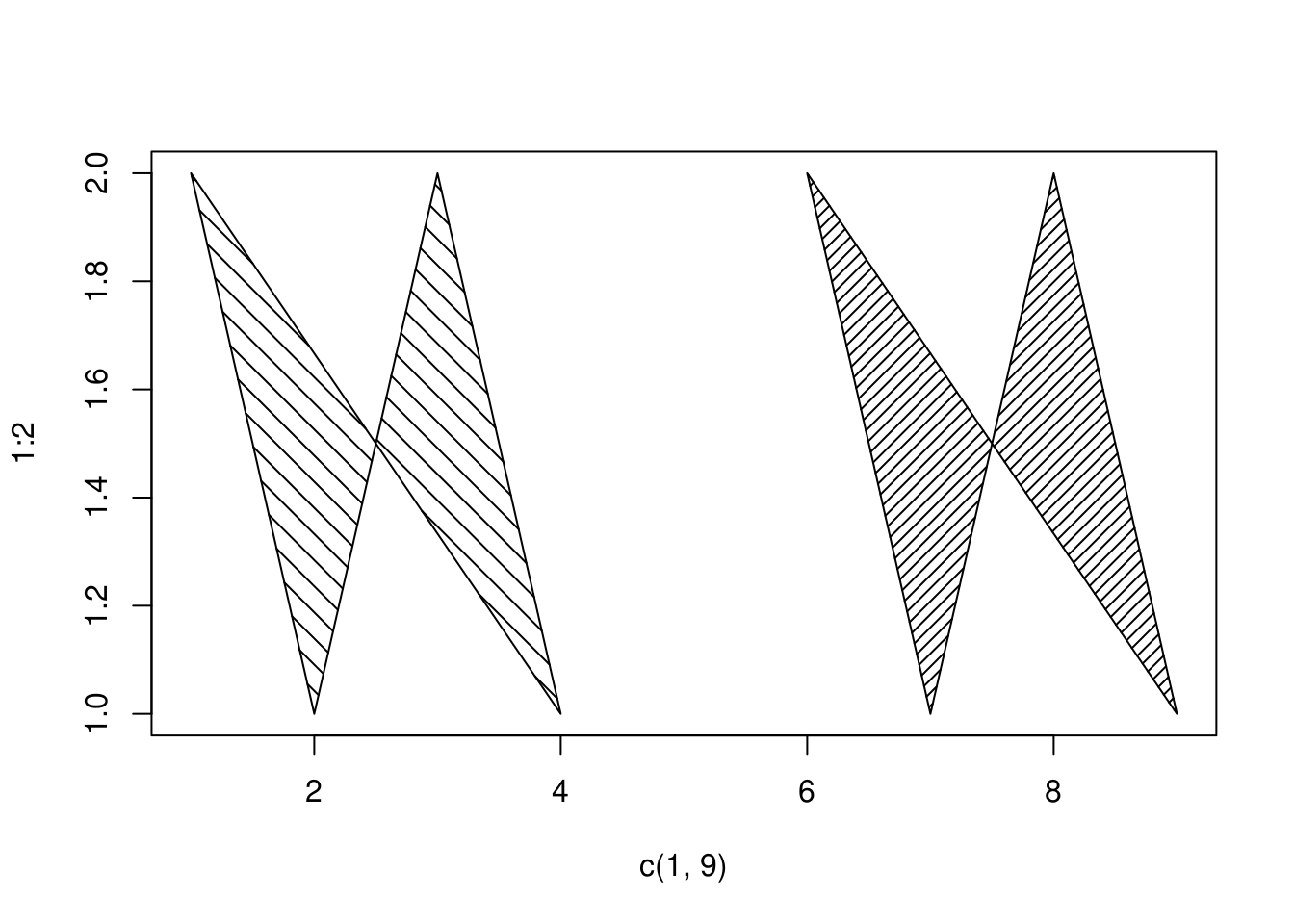R 画多边形，主要是 polygon() 函数。

## polygon 作图基本语法

polygon() 组图非常简单，直接提供 x/y 它就根据这一系列坐标为边界围成一个多边形。先直接看例子：

set.seed(1234)
x <- c(1:10, 10:1)
y <- c(rnorm(10, mean = 3), rnorm(10, mean = 5))
data.frame(x, y)
##     x         y
## 1   1 1.7929343
## 2   2 3.2774292
## 3   3 4.0844412
## 4   4 0.6543023
## 5   5 3.4291247
## 6   6 3.5060559
## 7   7 2.4252600
## 8   8 2.4533681
## 9   9 2.4355480
## 10 10 2.1099622
## 11 10 4.5228073
## 12  9 4.0016136
## 13  8 4.2237461
## 14  7 5.0644588
## 15  6 5.9594941
## 16  5 4.8897145
## 17  4 4.4889905
## 18  3 4.0888046
## 19  2 4.1628283
## 20  1 7.4158352

plot(1:10, 1:10, type = 'n',
ylim = c(1, 8),
xlab = "", ylab = "")
polygon(x, y, lwd = 2, col = 'green', border = "red")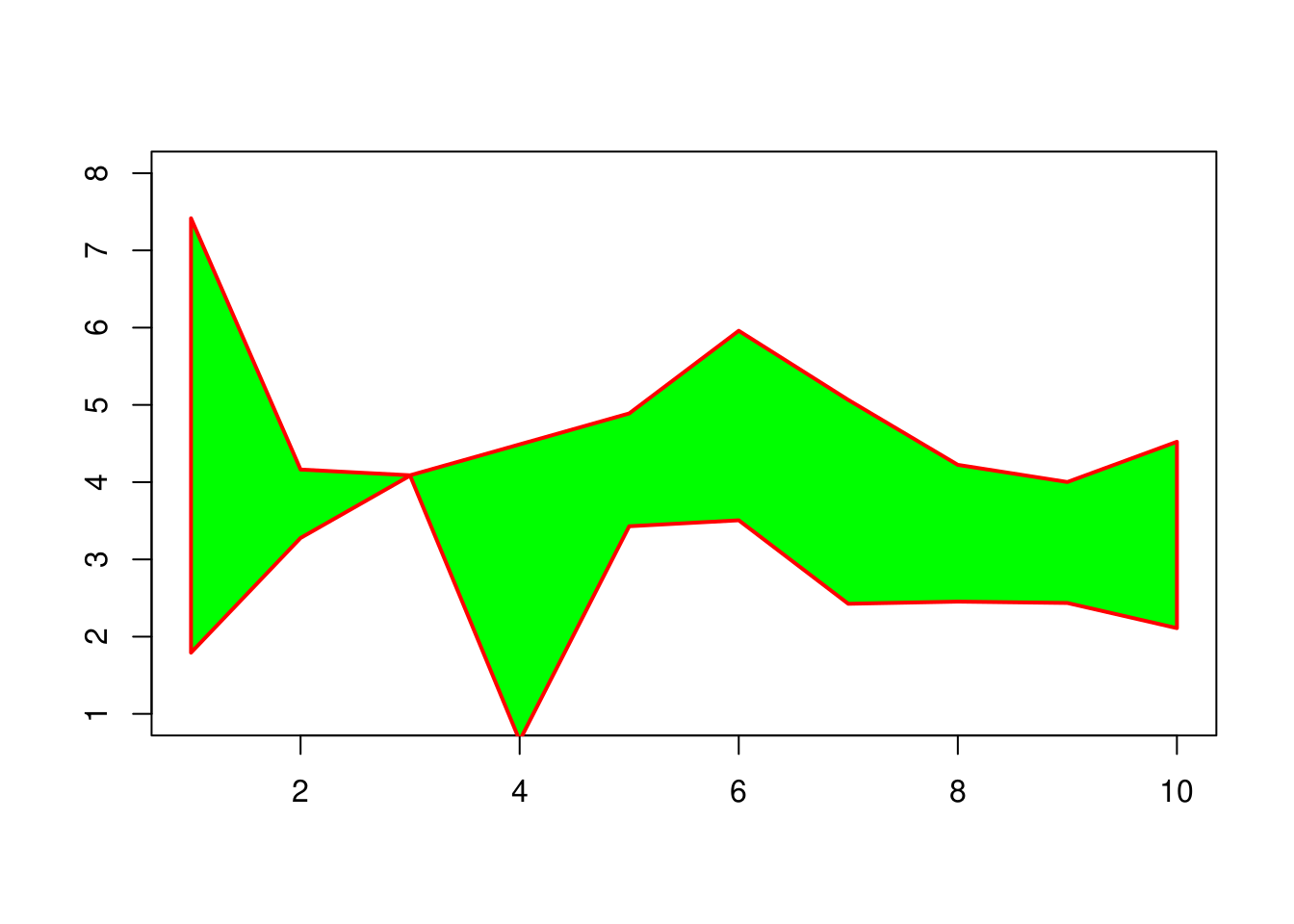x <- c(1:10, 9:2)
y <- c(rnorm(10, mean = 3), rnorm(8, mean = 5))
plot(1:10, 1:10, type = 'n',
ylim = c(1, 8),
xlab = "", ylab = "")
polygon(x, y, lwd = 3,
col = "red",
border = "green",
lty = "dashed")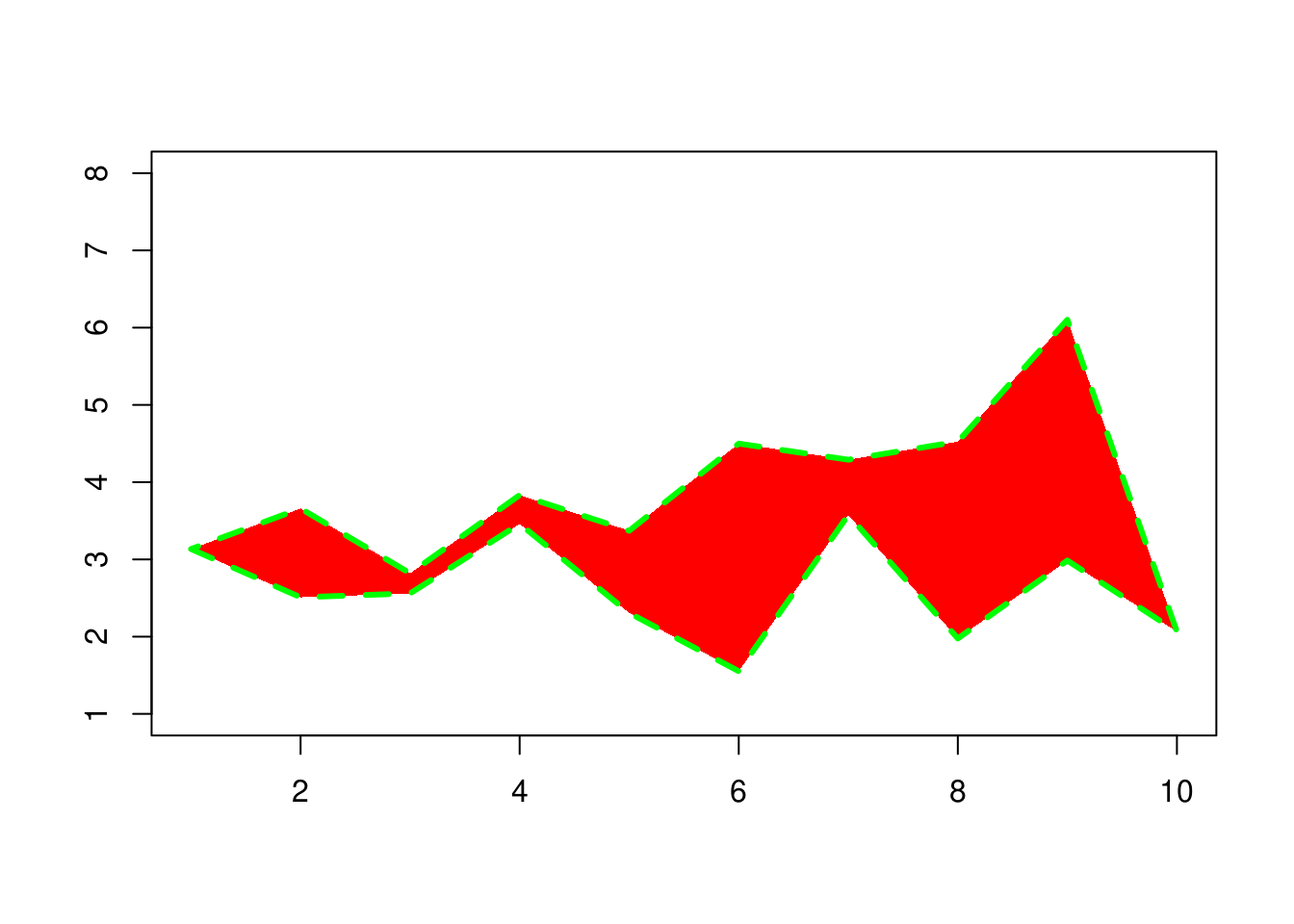## 文档示例

x <- c(1:9, 8:1)
y <- c(1, 2*(5:3), 2, -1, 17, 9, 8, 2:9)
op <- par(mfcol = c(3, 1))
for(xpd in c(FALSE, TRUE, NA)) {
plot(1:10, main = paste("xpd =", xpd))
box("figure", col = "pink", lwd = 3)
polygon(x, y,
xpd = xpd,
lty = 2, lwd = 2,
col = "orange", border = "red")
}
par(op)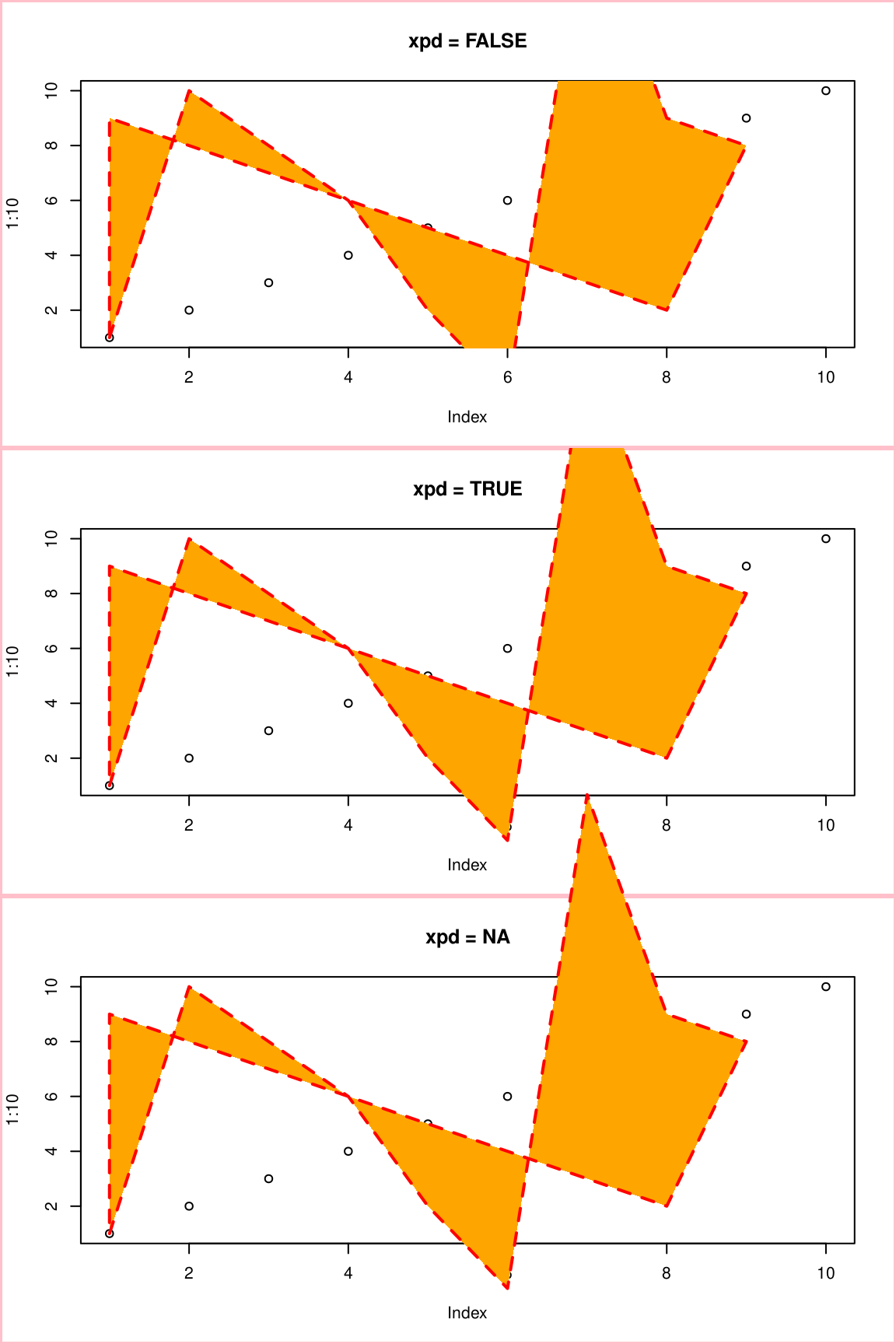n <- 100
xx <- c(0:n, n:0)
yy <- c(c(0, cumsum(stats::rnorm(n))),
rev(c(0, cumsum(stats::rnorm(n)))))
plot(xx, yy, type = "n", xlab = "Time", ylab = "Distance")
polygon(xx, yy, col = "gray", border = "red")
title("Distance Between Brownian Motions")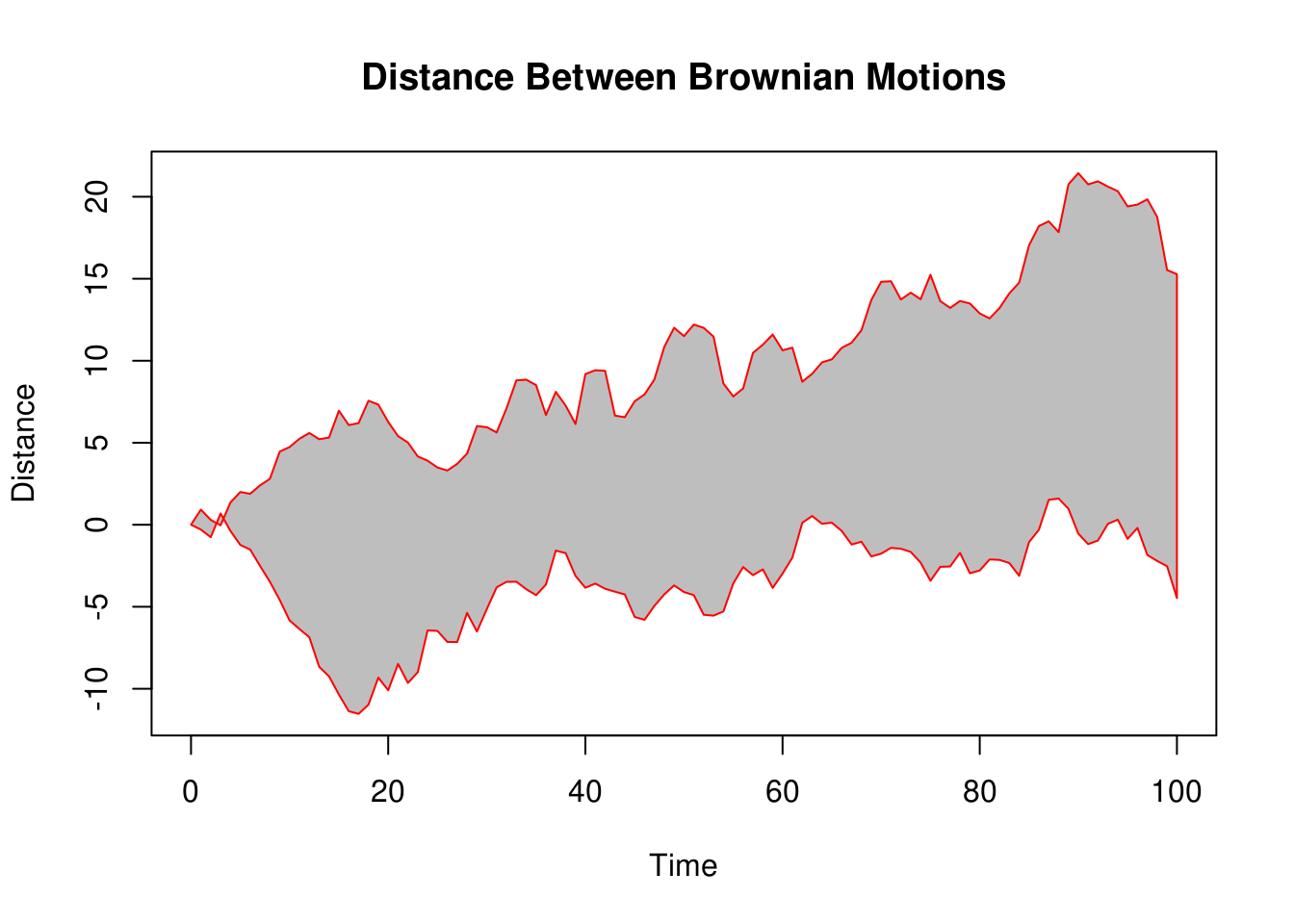op <- par(mfrow = c(2, 1))
plot(c(1, 9), 1:2, type = "n")
polygon(1:9, c(2, 1, 2, 1, 1, 2, 1, 2, 1),
col = c("red", "blue"),
border = c("green", "black"),
lwd = 3, lty = c("dashed", "solid"))
plot(c(1, 9), 1:2, type = "n")
polygon(1:9, c(2, 1, 2, 1, NA, 2, 1, 2, 1),
col = c("red", "blue"),
border = c("green", "black"),
lwd = 3, lty = c("dashed", "solid"))
par(op)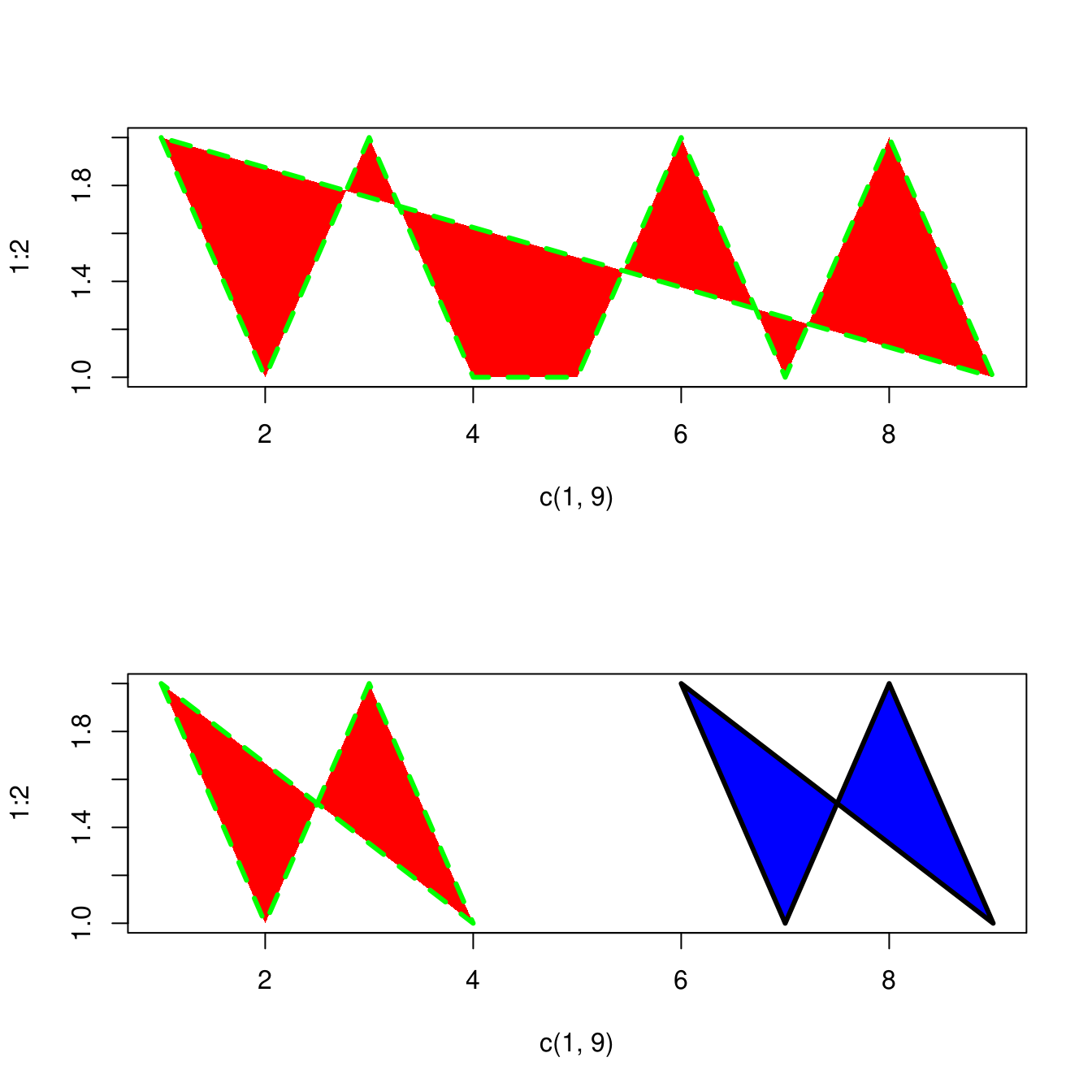plot(c(1, 9), 1:2, type = "n")
polygon(1:9,
c(2, 1, 2, 1, NA, 2, 1, 2, 1),
density = c(10, 20),
angle = c(-45, 45))